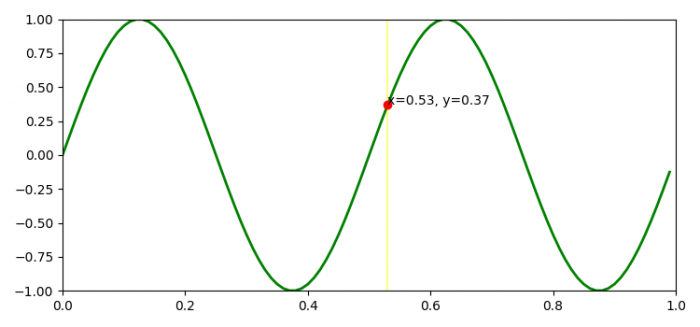# How to add a cursor to a curve in Matplotlib?

To add a cursor to a curve in Matplotlib, we can take the following steps −

• Set the figure size and adjust the padding between and around the subplots.
• Create t and s data points using numpy.
• Create a figure and a set of subplots.
• Get the cursor class instance, to update the cursor points on the plot.
• In mouse_event, get the x and y data of the current position of the mouse.
• Get the x and y data points' indices.
• Set the x and y positions.
• Set the text position and redraw agg buffer and mouse event.
• Plot t and s data points using plot() method.
• Set some axis properties.
• To display the figure, use show() method.

## Example

import matplotlib.pyplot as plt
import numpy as np

plt.rcParams["figure.figsize"] = [7.50, 3.50]
plt.rcParams["figure.autolayout"] = True

class CursorClass(object):
def __init__(self, ax, x, y):
self.ax = ax
self.ly = ax.axvline(color='yellow', alpha=0.5)
self.marker, = ax.plot(, , marker="o", color="red", zorder=3)
self.x = x
self.y = y
self.txt = ax.text(0.7, 0.9, '')

def mouse_event(self, event):
if event.inaxes:
x, y = event.xdata, event.ydata
indx = np.searchsorted(self.x, [x])
x = self.x[indx]
y = self.y[indx]
self.ly.set_xdata(x)
self.marker.set_data([x], [y])
self.txt.set_text('x=%1.2f, y=%1.2f' % (x, y))
self.txt.set_position((x, y))
self.ax.figure.canvas.draw_idle()
else:
return

t = np.arange(0.0, 1.0, 0.01)
s = np.sin(2 * 2 * np.pi * t)

fig, ax = plt.subplots()

cursor = CursorClass(ax, t, s)
cid = plt.connect('motion_notify_event', cursor.mouse_event)

ax.plot(t, s, lw=2, color='green')
plt.axis([0, 1, -1, 1])

plt.show()

## Output